# Kotlin Operators

In this tutorial you will learn about the Kotlin Operators and its application with practical example.

## Kotlin Operators

An operator is a special symbol that is used to carry out some specific operation on its operand. In Kotlin, we have rich set of built in operators to carry out different type of operations. There are operators for assignment, arithmetic operations, logical operations and comparison operations etc. Kotlin operators can be used with many types of variables or constants, but some of the operators are restricted to work on specific data types. Most operators are binary, meaning they take two operands, but a few are unary and only take one operand.

## Type of operators in Kotlin

Kotlin supports the following types of operators –

• Arithmetic Operators
• Assignment Operators
• Comparison (Relational) Operators
• Logical Operators
• Unary Operators
• Bitwise Operators

## Arithmetic Operators In Kotlin

Arithmetic Operators are used to perform arithmetic operations like addition, subtraction, multiplication, division, %modulus, exponent, etc.

Arithmetic operators in Kotlin
Operator Description Expression Translate to
`+` `Addition` `a+b` `a.plus(b)`
`-` `Subtraction` `a-b` `a.minus(b)`
`*` `Multiply` `a*b` `a.times(b)`
`/` `Division` `a/b` `a.div(b)`
`%` `Modulus` `a%b` `a.rem(b)`

Example:-

Output:-

## Assignment Operators In Kotlin

Assignment operators are used to assign value to a variable, you can assign a variable value or the result of an arithmetical expression.

Assignment operators in Kotlin
Operator Description Expression Convert to
`+=` `add and assign` `a+=b` `a.plusAssign(b)`
`-=` `subtract and assign` `a-=b` `a.minusAssign(b)`
`*=` `multiply and assign` `a*=b` `a.timesAssign(b)`
`/=` `divide and assign` `a/=b` `a.divAssign(b)`
`%=` `mod and assign` `a%=b` `a.remAssign(b)`

Example:-

Output:-## Comparison (Relational) Operators In Kotlin

Comparison Operators are used evaluate a comparison between two operands. The result of a comparison operation is a Boolean value that can only be true or false. Comparison Operators are also referred as relational operators.

Here is full list of Relational operators available in Kotlin –

Relational operators in Kotlin
Operator Description Expression Translate to
`>` `greater than` `a>b` `a.compateTo(b)>0`
`<` `Less than` `a<b` `a.compateTo(b)<0`
`>=` `greater than or equal to` `a>=b` `a.compateTo(b)>=0`
`<=` `less than or equal to` `a<=b` `a?.equals(b)?:(b===null)`
`==` `is equal to` `a==b` `a?.equals(b)?:(b===null)`
`!=` `not equal to` `a!=b` `!(a?.equals(b)?:(b===null))`

Example:-

Output:-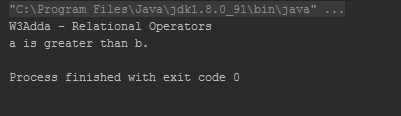## Kotlin Logical Operators

Logical operators are used to combine expressions with conditional statements using logical (AND,OR,NOT) operators, which results in true or false.

There are 3 logical operators available in Kotlin.

Logical operators in Kotlin
Operator Description Expression Convert to
`&&` `return true if all expression are true` `(a>b) && (a>c)` `(a>b) and (a>c)`
`||` `return true if any expression are true` `(a>b) || (a>c)` `(a>b) or(a>c)`
`!` `return complement of expression` `!a` `a.not()`

Example:-

Output:-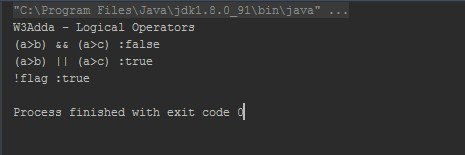## Unary Operators In Kotlin

The unary operators operate on a single operand, here is full list of kotlin unary operators.

Unary operators in Kotlin
Operator Description Expression Convert to
`+` `unary plus` `+a` `a.unaryPlus()`
`-` `unary minus` `-a` `a.unaryMinus()`
`++` `increment by 1` `++a` `a.inc()`
`--` `decrement by 1` `--a` `a.dec()`
`!` `not` `!a` `a.not()`

Example:-

Output:-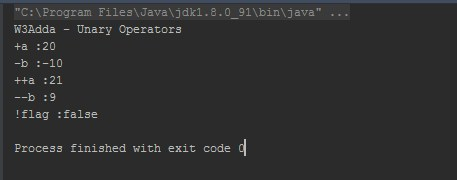## Bitwise operators in Kotlin

Bitwise operator are used to perform bit level operation over its operand.

Bitwise operators in Kotlin
Named Function Description Expression
`shl (bits)` `signed shift left` `a.shl(b)`
`shr (bits)` `signed shift right` `a.shr(b)`
`ushr (bits)` `unsigned shift right` `a.ushr(b)`
`and (bits)` `bitwise and` `a.and(b)`
`or (bits)` `bitwise or` `a.or(b)`
`xor (bits)` `bitwise xor` `a.xor(b)`
`inv()` `bitwise inverse` `a.inv()`

Example:-

Output:-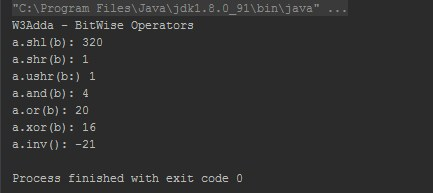## Kotlin in Operator

Kotlin in operator allows you to check whether an object belongs to a collection or not.

Kotlin in Operator
Operator Expression Translates to
`in` `a in b` `b.contains(a)`
`!in` `a !in b` `!b.contains(a)`

Example:-

Output:-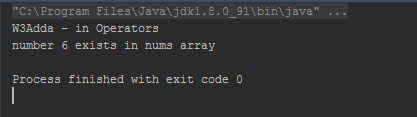## Index access Operator

Index access operator allows you to access elements with specified index in an array or collection.

Kotlin Index access Operator
Expression Translated to
`a[i]` `a.get(i)`
`a[i, n]` `a.get(i, n)`
`a[i1, i2, ..., in]` `a.get(i1, i2, ..., in)`
`a[i] = b` `a.set(i, b)`
`a[i, n] = b` `a.set(i, n, b)`
`a[i1, i2, ..., in] = b` `a.set(i1, i2, ..., in, b)`

Example:-

Output:-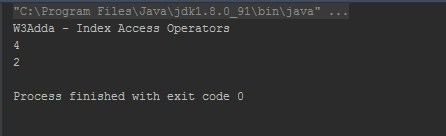## Kotlin Invoke Operator

Kotlin Invoke Operator
Expression Translated to
`a()` `a.invoke()`
`a(i)` `a.invoke(i)`
`a(i1, i2, ..., in)` `a.inkove(i1, i2, ..., in)`
`a[i] = b` `a.set(i, b)`

In this tutorial we have learn about the Kotlin Operators and its application with practical example. I hope you will like this tutorial.